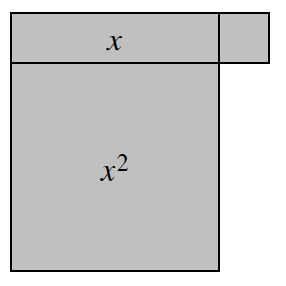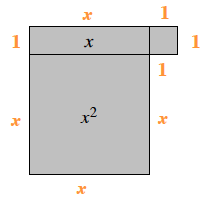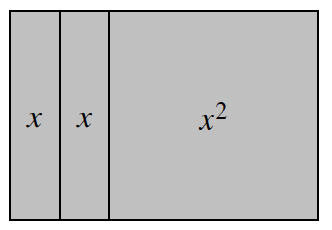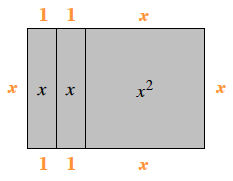### Home > CC1 > Chapter 6 > Lesson 6.2.3 > Problem6-96

6-96.

Copy the diagrams of algebra tiles below on your paper. Then find the perimeter of each shape.

1.The perimeter is the sum of all the sides.
What is the value of each side?

Now, add up all the sides to find the perimeter.
$x+1+x+1+1+1+x+x$Simplify, and the perimeter is $4x+4$.

1.Refer to part (a).